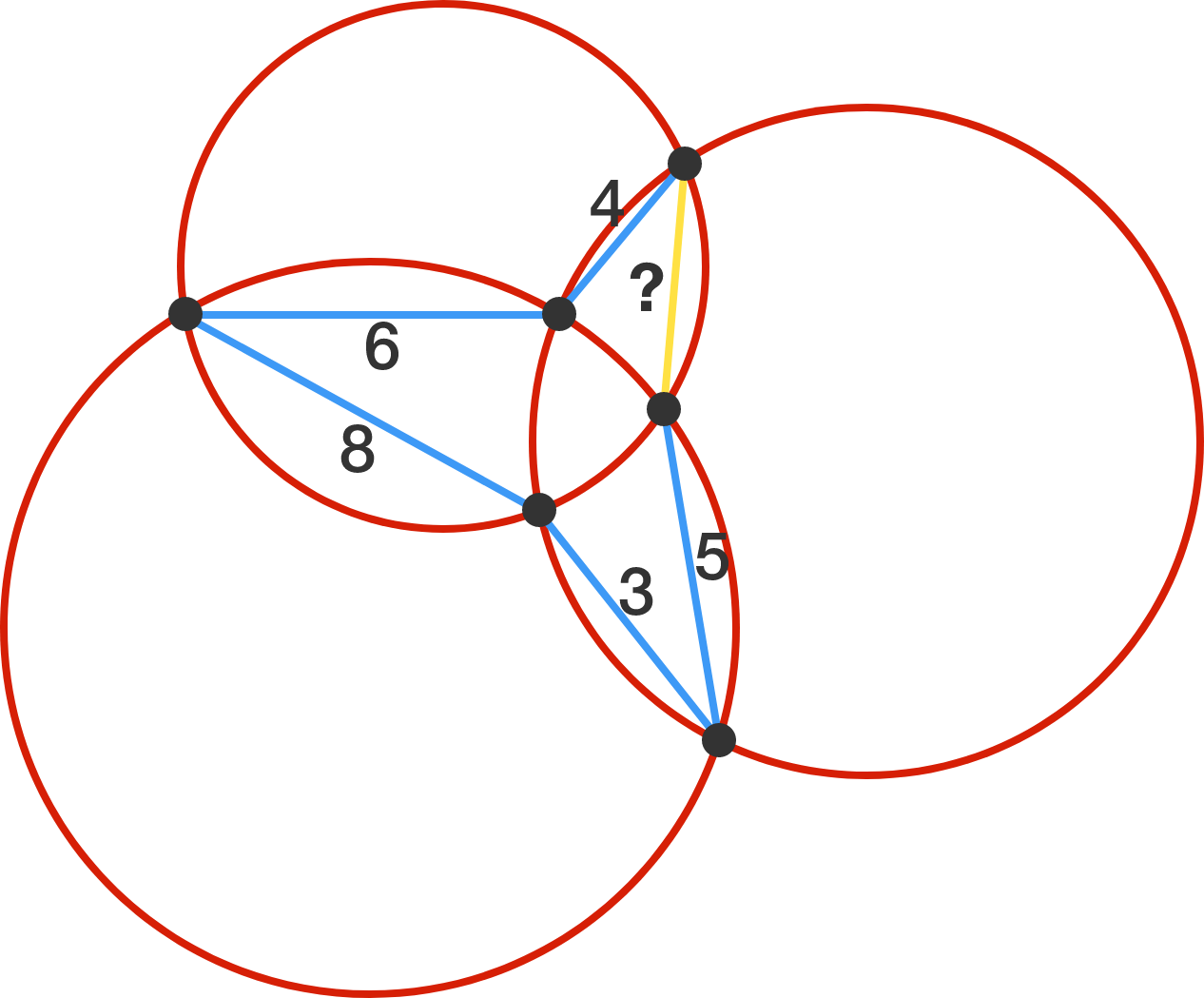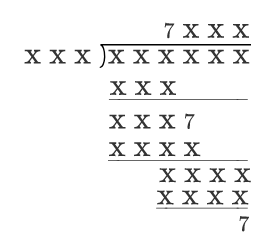# Problems of the Week

Contribute a problem

# 2017-07-17 Advanced

If $N$ is divisible by $1,2,3,\ldots, 13$, then $N$ must also be divisible by 14 and 15.

Using this same idea, what is the smallest integer $M$ such that the following statement is true?

If $N$ is divisible by $1,2,3,\ldots,M$, then $N$ must also be divisible by $M+1,M+2,M+3,$ and $M+4$.

Suppose there exists a triangle with integer side lengths such that its perimeter (in units) and area (in square units) have the same numerical value, $p$.

What is the sum of all possible values of $p?$

As shown in the diagram below, three overlapping circles with distinct radii define six points. The lengths of 5 line segments (in blue) connecting the points are known to us: 4, 6, 8, 3, 5.

What is the length of the remaining segment (in yellow), to 3 decimal places?The above is a long division with most of the digits of any number hidden, except for the three 7's. Given that each of 0, 1, 2, ..., 9 was used at least once for the hidden digits, figure out all of the digits hiding and submit your answer as the value of the dividend (the 6-digit number being divided).

Details and Assumptions:

• Each $\mathrm X$ represents a single-digit integer.
• The leading (leftmost) digit of a number cannot be 0.

Find all integer solutions to the equation $y^2=x^3-p^3$, where $p$ is a prime number, $y \neq 0$, $3 \nmid y$, and $p \nmid y$. Enter your answer as $\sum(x+p)$.

Note: Actually, you are going to find integer points on a family of Mordell curves. A Mordell curve is an elliptic curve of the form $y^2 = x^3 + k$, where $k$ is a fixed non-zero integer.

×

Problem Loading...

Note Loading...

Set Loading...